Analytics Vidhya — February 28, 2017

## Introduction

Optimization is the way of life. We all have finite resources and time and we want to make the most of them. From using your time productively to solving supply chain problems for your company – everything uses optimization. It’s an especially interesting and relevant topic in data science.

It is also a very interesting topic – it starts with simple problems, but it can get very complex. For example, sharing a bar of chocolate between siblings is a simple optimization problem. We don’t think in mathematical terms while solving it. On the other hand, devising inventory and warehousing strategy for an e-tailer can be very complex. Millions of SKUs with different popularity in different regions to be delivered in defined time and resources – you see what I mean!

Linear programming (LP) is one of the simplest ways to perform optimization. It helps you solve some very complex optimization problems by making a few simplifying assumptions. As an analyst, you are bound to come across applications and problems to be solved by Linear Programming.

For some reason, LP doesn’t get as much attention as it deserves while learning data science. So, I thought let me do justice to this awesome technique. I decided to write an article that explains Linear programming in simple English. I have kept the content as simple as possible. The idea is to get you started and excited about Linear Programming.

Note- If you want to learn this in a course format, we have curated this free course for you- Linear Programming for Data Science Professionals

## Table of Content

1. What is Linear Programming?
• Basic Terminologies
• The process to define an LP problem
2. Solve Linear Program by Graphical Method
3. Solve Linear Program using R
4. Solve Linear Program using OpenSolver
5. Simplex Method
6. Northwest Corner Method and Least Cost Method
7. Applications of Linear programming

## 1. What is Linear Programming?

Now, what is linear programming? Linear programming is a simple technique where we depict complex relationships through linear functions and then find the optimum points. The important word in the previous sentence is depicted. The real relationships might be much more complex – but we can simplify them to linear relationships.

Applications of linear programming are everywhere around you. You use linear programming at personal and professional fronts. You are using linear programming when you are driving from home to work and want to take the shortest route. Or when you have a project delivery you make strategies to make your team work efficiently for on-time delivery.

### Example of a linear programming problem

Let’s say a FedEx delivery man has 6 packages to deliver in a day. The warehouse is located at point A. The 6 delivery destinations are given by U, V, W, X, Y, and Z. The numbers on the lines indicate the distance between the cities. To save on fuel and time the delivery person wants to take the shortest route.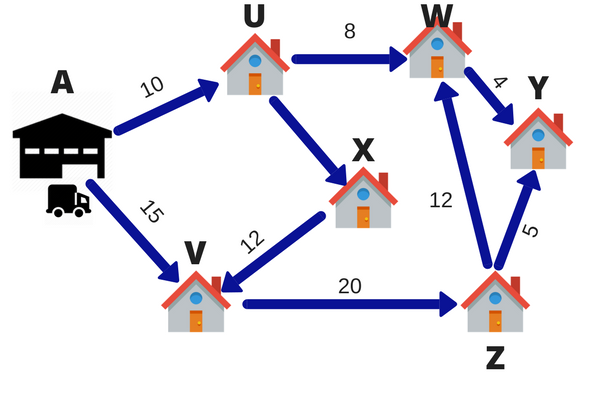So, the delivery person will calculate different routes for going to all the 6 destinations and then come up with the shortest route. This technique of choosing the shortest route is called linear programming.

In this case, the objective of the delivery person is to deliver the parcel on time at all 6 destinations. The process of choosing the best route is called Operation Research. Operation research is an approach to decision-making, which involves a set of methods to operate a system. In the above example, my system was the Delivery model.

Linear programming is used for obtaining the most optimal solution for a problem with given constraints. In linear programming, we formulate our real-life problem into a mathematical model. It involves an objective function, linear inequalities with subject to constraints.

Is the linear representation of the 6 points above representative of the real-world? Yes and No. It is an oversimplification as the real route would not be a straight line. It would likely have multiple turns, U-turns, signals and traffic jams. But with a simple assumption, we have reduced the complexity of the problem drastically and are creating a solution that should work in most scenarios.

### Formulating a problem – Let’s manufacture some chocolates

Example: Consider a chocolate manufacturing company that produces only two types of chocolate – A and B. Both the chocolates require Milk and Choco only.  To manufacture each unit of A and B, the following quantities are required:

• Each unit of A requires 1 unit of Milk and 3 units of Choco
• Each unit of B requires 1 unit of Milk and 2 units of Choco

The company kitchen has a total of 5 units of Milk and 12 units of Choco. On each sale, the company makes a profit of

• Rs 6 per unit A sold
• Rs 5 per unit B sold.

Now, the company wishes to maximize its profit. How many units of A and B should it produce respectively?

Solution: The first thing I’m gonna do is represent the problem in a tabular form for better understanding.

 Milk Choco Profit per unit A 1 3 Rs 6 B 1 2 Rs 5 Total 5 12

Let the total number of units produced by A be = X

Let the total number of units produced by B be = Y

Now, the total profit is represented by Z

The total profit the company makes is given by the total number of units of A and B produced multiplied by its per-unit profit of Rs 6 and Rs 5 respectively.

Profit: Max Z = 6X+5Y

which means we have to maximize Z.

The company will try to produce as many units of A and B to maximize the profit. But the resources Milk and Choco are available in a limited amount.

As per the above table, each unit of A and B requires 1 unit of Milk. The total amount of Milk available is 5 units. To represent this mathematically,

X+Y ≤ 5

Also, each unit of A and B requires 3 units & 2 units of Choco respectively. The total amount of Choco available is 12 units. To represent this mathematically,

3X+2Y ≤ 12

Also, the values for units of A can only be integers.

So we have two more constraints, X ≥ 0  &  Y ≥ 0

For the company to make maximum profit, the above inequalities have to be satisfied.

This is called formulating a real-world problem into a mathematical model.

### Common terminologies used in Linear Programming

Let us define some terminologies used in Linear Programming using the above example.

• Decision Variables: The decision variables are the variables that will decide my output. They represent my ultimate solution. To solve any problem, we first need to identify the decision variables. For the above example, the total number of units for A and B denoted by X & Y respectively are my decision variables.
• Objective Function: It is defined as the objective of making decisions. In the above example, the company wishes to increase the total profit represented by Z. So, profit is my objective function.
• Constraints: The constraints are the restrictions or limitations on the decision variables. They usually limit the value of the decision variables. In the above example, the limit on the availability of resources Milk and Choco are my constraints.
• Non-negativity restriction: For all linear programs, the decision variables should always take non-negative values. This means the values for decision variables should be greater than or equal to 0.

### The process to formulate a Linear Programming problem

Let us look at the steps of defining a Linear Programming problem generically:

1. Identify the decision variables
2. Write the objective function
3. Mention the constraints
4. Explicitly state the non-negativity restriction

For a problem to be a linear programming problem, the decision variables, objective function and constraints all have to be linear functions.

If all the three conditions are satisfied, it is called a Linear Programming Problem.

## 2. Solve Linear Programs by Graphical Method

A linear program can be solved by multiple methods. In this section, we are going to look at the Graphical method for solving a linear program. This method is used to solve a two-variable linear program. If you have only two decision variables, you should use the graphical method to find the optimal solution.

A graphical method involves formulating a set of linear inequalities subject to the constraints. Then the inequalities are plotted on an X-Y plane. Once we have plotted all the inequalities on a graph the intersecting region gives us a feasible region. The feasible region explains what all values our model can take. And it also gives us the optimal solution.

Let’s understand this with the help of an example.

Example: A farmer has recently acquired a 110 hectares piece of land. He has decided to grow Wheat and barley on that land. Due to the quality of the sun and the region’s excellent climate, the entire production of Wheat and Barley can be sold. He wants to know how to plant each variety in the 110 hectares, given the costs, net profits and labor requirements according to the data shown below:

 Variety Cost (Price/Hec) Net Profit (Price/Hec) Man-days/Hec Wheat 100 50 10 Barley 200 120 30

The farmer has a budget of US\$10,000 and availability of 1,200 man-days during the planning horizon. Find the optimal solution and the optimal value.

Solution: To solve this problem, first we gonna formulate our linear program.

#### Formulation of Linear Problem

Step 1: Identify the decision variables

The total area for growing Wheat = X (in hectares)

The total area for growing Barley  = Y (in hectares)

X and Y are my decision variables.

Step 2: Write the objective function

Since the production from the entire land can be sold in the market. The farmer would want to maximize the profit for his total produce. We are given net profit for both Wheat and Barley. The farmer earns a net profit of US\$50 for each hectare of Wheat and US\$120 for each Barley.

Our objective function (given by Z) is, Max Z = 50X + 120Y

Step 3: Writing the constraints

1. It is given that the farmer has a total budget of US\$10,000. The cost of producing Wheat and Barley per hectare is also given to us. We have an upper cap on the total cost spent by the farmer. So our equation becomes:

100X + 200Y ≤ 10,000

2. The next constraint is the upper cap on the availability of the total number of man-days for the planning horizon. The total number of man-days available is 1200. As per the table, we are given the man-days per hectare for Wheat and Barley.

10X + 30Y ≤ 1200

3. The third constraint is the total area present for plantation. The total available area is 110 hectares. So the equation becomes,

X + Y ≤ 110

Step 4: The non-negativity restriction

The values of X and Y will be greater than or equal to 0. This goes without saying.

X ≥ 0, Y ≥ 0

We have formulated our linear program. It’s time to solve it.

#### Solving an LP through Graphical method

Since we know that X, Y ≥ 0. We will consider only the first quadrant.

To plot for the graph for the above equations, first I will simplify all the equations.

100X + 200Y ≤ 10,000 can be simplified to X + 2Y ≤ 100 by dividing by 100.

10X + 30Y ≤ 1200 can be simplified to X + 3Y ≤ 120 by dividing by 10.

The third equation is in its simplified form, X + Y ≤ 110.

Plot the first 2 lines on a graph in the first quadrant (like shown below)

The optimal feasible solution is achieved at the point of intersection where the budget & man-days constraints are active. This means the point at which the equations X + 2Y ≤ 100 and X + 3Y ≤ 120 intersect gives us the optimal solution.

The values for X and Y which gives the optimal solution is at (60,20).

To maximize profit the farmer should produce Wheat and Barley in 60 hectares and 20 hectares of land respectively.

The maximum profit the company will gain is,

Max Z = 50 * (60) + 120 * (20)

=  US\$5400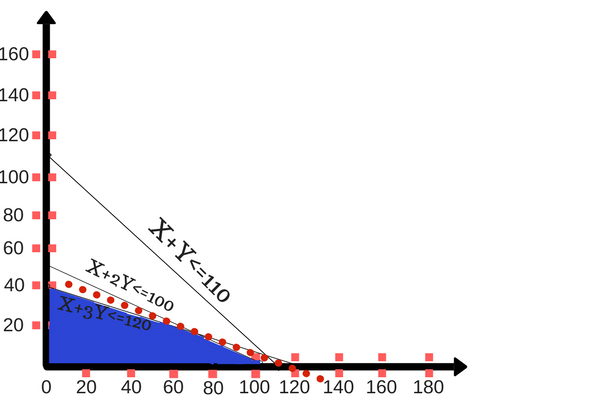Note: Everything taught here has also been taught in a course format in this free course- Linear Programming for Data Science Professionals

## 3. Solve Linear Program Using R

R is an open-source tool that is very popular among the data scientists for essential data science tasks. Performing linear programming is very easy and we can attain an optimum solution in very few steps. Come let’s learn.

Example: A toy manufacturing organization manufactures two types of toys A and B. Both the toys are sold at Rs.25 and Rs.20 respectively. There are 2000 resource units available every day from which the toy A requires 20 units while toy B requires 12 units. Both of these toys require a production time of 5 minutes. Total working hours are 9 hours a day. What should be the manufacturing quantity for each of the pipes to maximize the profits?

Here:

The objective function is:
Max.Z=25x+20y

where x are the units of pipe A

y are the units of pipe B

Constraints:
20x+12y<=2000

5x+5y<=540

Let’s see the code part now:

### Output

```summary(opt)

Length Class Mode
direction 1 -none- numeric
x.count 1 -none- numeric
objective 2 -none- numeric
const.count 1 -none- numeric
constraints 8 -none- numeric
int.count 1 -none- numeric
int.vec 1 -none- numeric
bin.count 1 -none- numeric
binary.vec 1 -none- numeric
num.bin.solns 1 -none- numeric
objval 1 -none- numeric
solution 2 -none- numeric
presolve 1 -none- numeric
compute.sens 1 -none- numeric
sens.coef.from 1 -none- numeric
sens.coef.to 1 -none- numeric
duals 1 -none- numeric
duals.from 1 -none- numeric
duals.to 1 -none- numeric
scale 1 -none- numeric
use.dense 1 -none- numeric
dense.col 1 -none- numeric
dense.val 1 -none- numeric
dense.const.nrow 1 -none- numeric
dense.ctr 1 -none- numeric
use.rw 1 -none- numeric
tmp 1 -none- character
status 1 -none- numeric

> opt\$solution
 88 20

> opt\$objval
 2600

```

Therefore from the output, we see that the organization should produce 88 units of toy A and 20 units of toy B and the maximum profit for the organization will be Rs.2600.

## 4. Solve Linear Program using OpenSolver

In reality, a linear program can contain 30 to 1000 variables and solving it either Graphically or Algebraically is next to impossible. Companies generally use OpenSolver to tackle these real-world problems. Here I am gonna take you through steps to solve a linear program using OpenSolver.

OpenSolver is an open-source linear and optimizer for Microsoft Excel. It is an advanced version of a built-in Excel Solver. You can download OpenSolver here and follow the installation manual.

I want you to get hands-on knowledge of using OpenSolver. So, for a clear understanding, I will explain it using an example.

Example: Below there is a diet chart that gives me calories, protein, carbohydrate and fat content for 4 food items. Sara wants a diet with minimum cost. The diet chart is as follows:

 Food Item 1 Food Item 2 Food Item 3 Food Item 4 Calories 400 200 150 500 Protien (in grams) 3 2 0 0 Carbohydrates ( in grams) 2 2 4 4 Fat (in grams) 2 4 1 5 Cost \$0.50 \$0.20 \$0.30 \$0.80

The chart gives the nutrient content as well as the per-unit cost of each food item. The diet has to be planned in such a way that it should contain at least 500 calories, 6 grams of protein, 10 grams of carbohydrates and 8 grams of fat.

Solution: First, I’m gonna formulate my linear program in a spreadsheet.

• Step 1: Identify the decision variables. Here my decision variables are the food items. Add the headers. For trial purposes, we are entering arbitrary values. Let’s say, Sara consumes 3 units of Food Item 1, 0 unit of Food Item 2, 1  unit of Food Item 3 and 0 unit of Food Item 4. These are called variable cells.• Step 2: Now we will write our objective function. For the diet to be optimal we must have minimum cost along with required calories, protein, carbohydrate, and fat.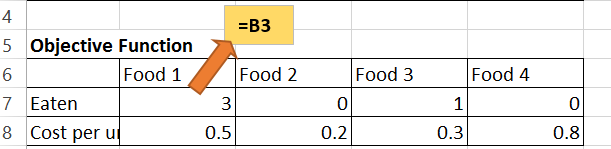In cell B7:E7  we take the reference to the number of units. And in cell B8:E8 we put the per-unit cost of each food item.

In cell B10, we want the total cost of the diet. The total cost is given by the sumproduct of the number of units eaten and per-unit cost. Sumproduct is given by = B7*B8+C7*C8+D7*D8+E7*E8. Let’s see this in a spreadsheet.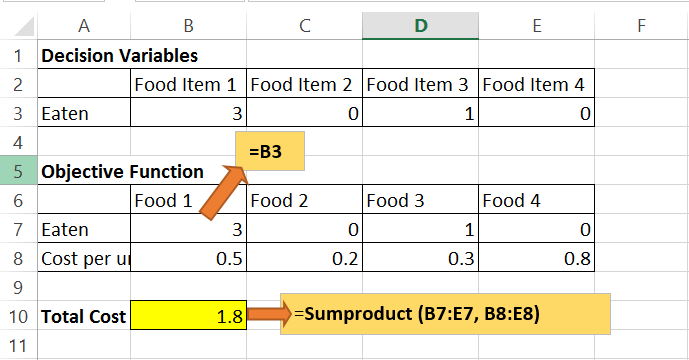• Step 3: Now, we will enter the constraints.  Column F contains a total of calories, protein, carbohydrate, and fat. The total number of calorie intake in given by sumproduct the number of food items eaten and the calorie consumed per food item. For cell F13= Sumprodcut(\$B\$7:\$F\$7, B13:F13). Similarly for others. Column G gives the inequality since the problem demands Calories, Protein, Carbohydrate and Fat to be at least 500, 6, 10 and 8 respectively. Column H gives the required nutrient content.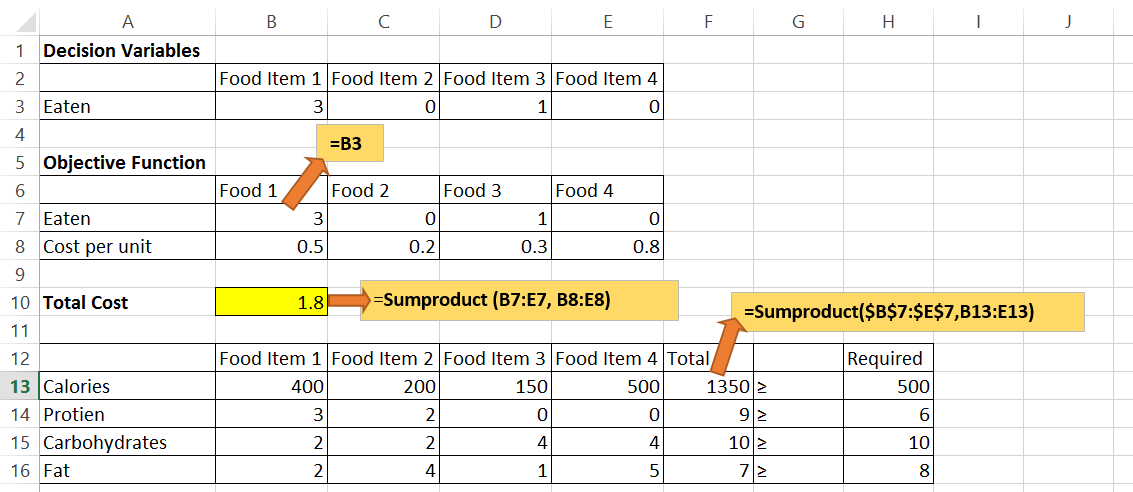• Step 4: Now, we will enter the Linear program into the solver. Now, once you have installed OpenSolver. When you click on the Data tab, on the right you will see Model. Click on the model, then enter the values one by one. First, we will enter the objective function,\$B10 i.e in the objective cell. Select minimize because we want to minimize the diet cost.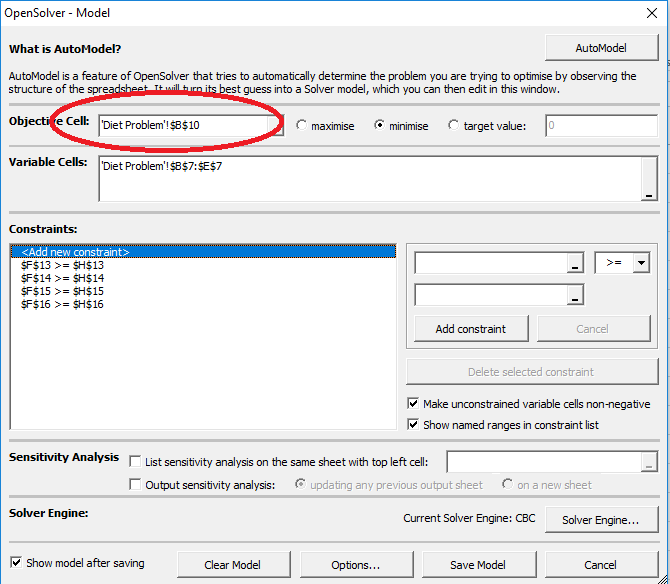• Step 5: Now enter the decision variables in the variable cells.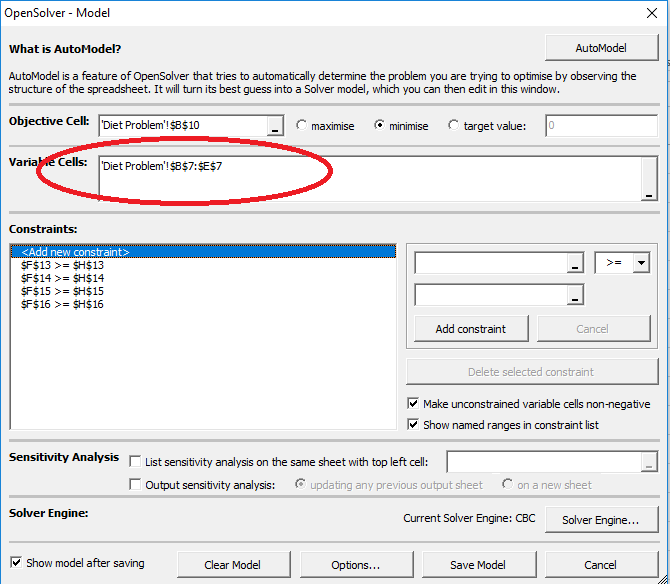• Step 6: Now, we will add the constraints. The first constraint is the F13 ≥  H13. Add all the constraints one by one.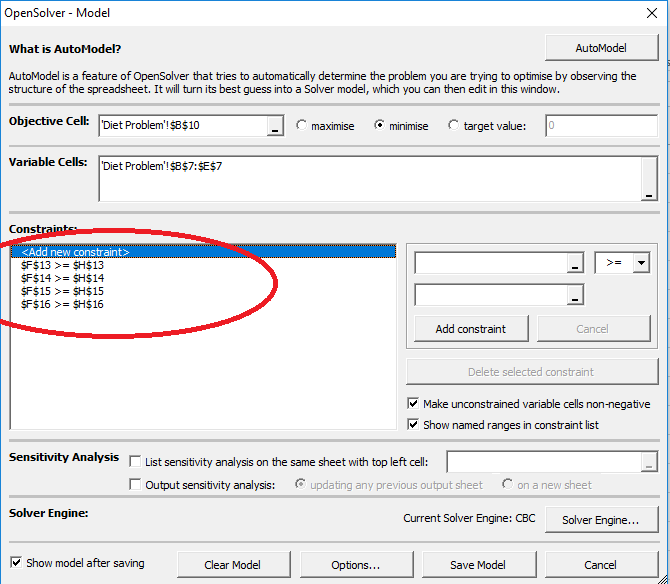• Step 7: Now, you have to enter one important constraint. The non-negativity restriction. All the decision variables will be greater than 0.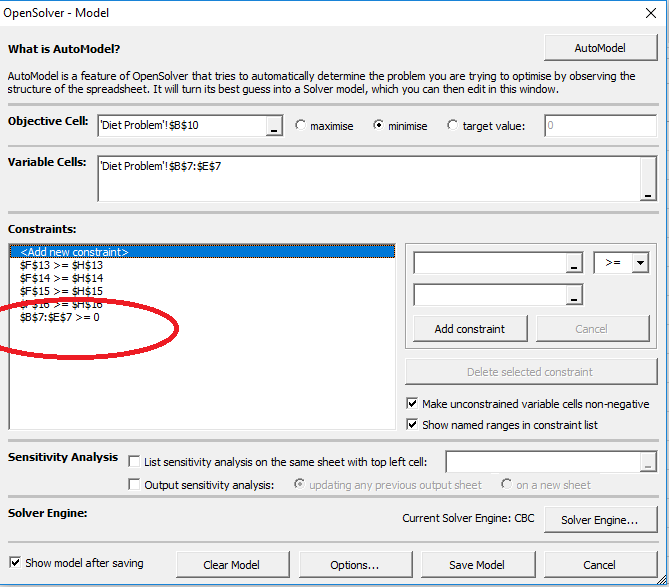• Step 8: Now, click on Save Model to finish the modeling process. Once you save the model, it will look something like this.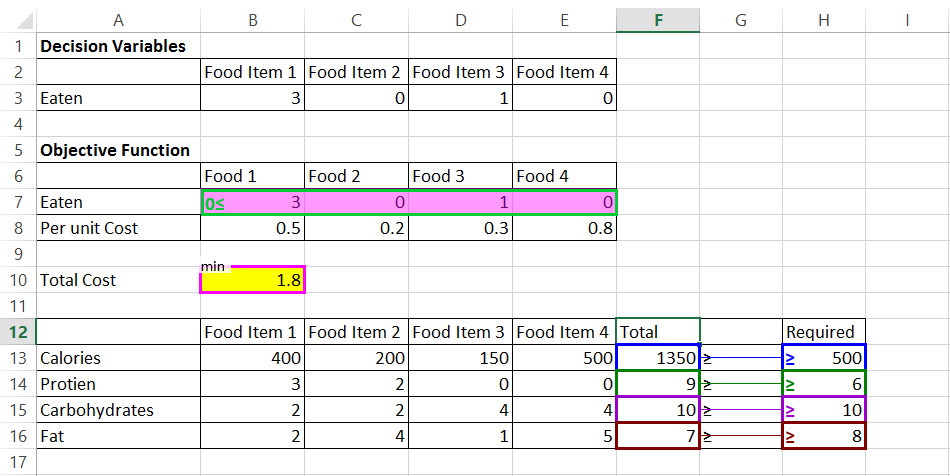• Step 9: Once the model is saved click on the Data tab then click solve. The optimal solution and values are displayed in the corresponding cells. The optimal minimum cost is US\$0.90. Sara should consume 3 units of Food Item 2 and 1 unit of Food Item 3 for the required nutrient content at the minimum cost. This solves our linear program.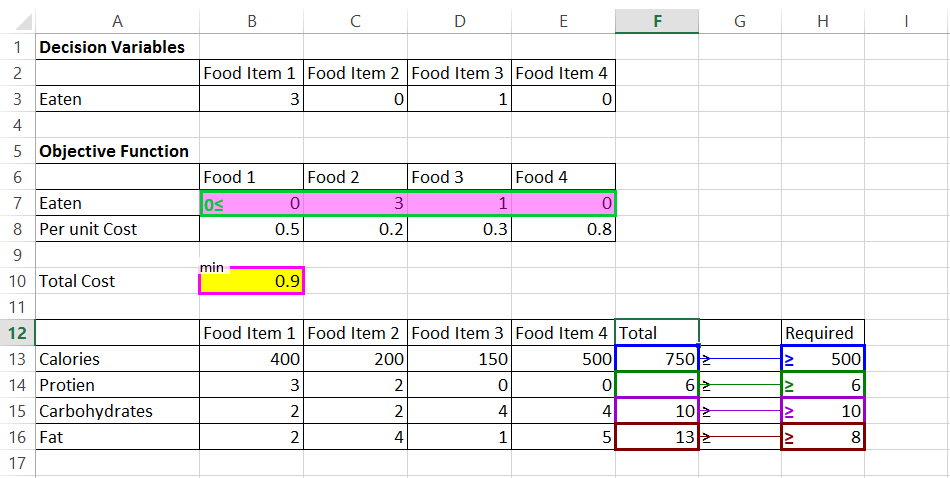## 5. Simplex Method

Simplex Method is one of the most powerful & popular methods for linear programming. The simplex method is an iterative procedure for getting the most feasible solution. In this method, we keep transforming the value of basic variables to get maximum value for the objective function.

A linear programming function is in its standard form if it seeks to maximize the objective function.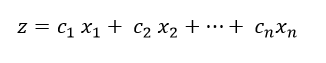subject to constraints,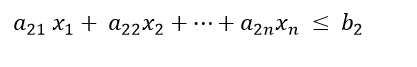.                 .                 .                 .                .              .

.                 .                 .                  .               .             .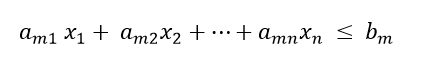where,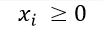and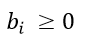. After adding slack variables, the corresponding system of constraint equation is,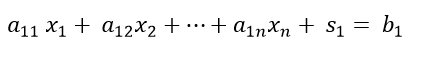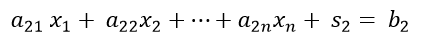.                                    .                                       .                    .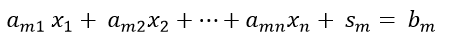where,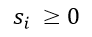The variables,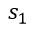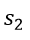……………….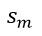are called slack variables. They are non-negative numbers that are added to remove the inequalities from an equation.

The above explanation gives the theoretical explanation of the simplex method. Now, I am gonna explain how to use the simplex method in real life using Excel.

Example: The advertising alternatives for a company include television, newspaper and radio advertisements. The cost for each medium with its audience coverage is given below.

The local newspaper limits the number of advertisements from a single company to ten. Moreover, in order to balance the advertising among the three types of media, no more than half of the total number of advertisements should occur on the radio. And at least 10% should occur on television. The weekly advertising budget is \$18,200. How many advertisements should be run in each of the three types of media to maximize the total audience?

Solution: First I am going to formulate my problem for a clear understanding.

Step 1: Identify Decision Variables

Let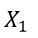,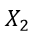,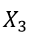represent the total number of ads for television, newspaper, and radio respectively.

Step 2: Objective Function

The objective of the company is to maximize the audience. The objective function is given by: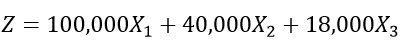Step 3: Write down the constraints

Now, I will mention each constraint one by one.

It is clearly given that we have a budget constraint. The total budget which can be allocated is \$18,200. And the individual costs per television, newspaper and radio advertisement is \$2000, \$600 and \$300 respectively. This can be represented by the equation,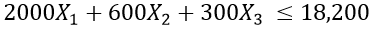For a newspaper advertisement, there is an upper cap on the number of advertisements to 10. My first constraints are,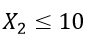The next constraint is the number of advertisements on television. The company wants at least 10% of the total advertisements to be on television. So, it can be represented as: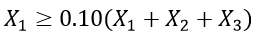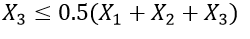Now, I have formulated my linear programming problem. We are using the simplex method to solve this. I will take you through the simplex method one by one.

To reiterate all the constraints are as follows. I have simplified the last two equations to bring them in standard form.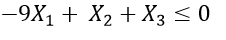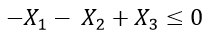We have a total of 4 equations. To balance out each equation, I am introducing 4 slack variables,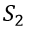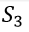and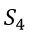.

So our equations are as follows: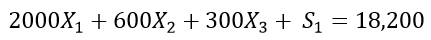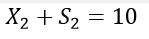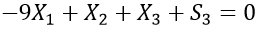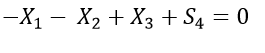I hope now you are available to make sense of the entire advertising problem. All the above equations are only for your better understanding. Now if you solve these equations, you will get the values for X1= 4, X2= 10 and X3= 14.

On solving the objective function you will get the maximum weekly audience as 1,052,000. You can follow the tutorial here to solve the equation. To solve a linear program in excel, follow this tutorial.

## 6. Northwest Corner Method and Least Cost Method

### 6.1 Northwest Corner Method

The northwest corner method is a special type method used for transportation problems in linear programming. It is used to calculate the feasible solution for transporting commodities from one place to another. Whenever you are given a real-world problem, which involves supply and demand from one source of a different sources. The data model includes the following:

• The level of supply and demand at each source is given
• The unit transportation of a commodity from each source to each destination

The model assumes that there is only one commodity. The demand for which can come from different sources. The objective is to fulfill the total demand with minimum transportation cost. The model is based on the hypothesis that the total demand is equal to the total supply, i.e the model is balanced. Let’s understand this with the help of an example.

Example: Consider there are 3 silos which are required to satisfy the demand from 4 mills. (A silo is a storage area of the farm used to store grain and Mill is a grinding factory for grains).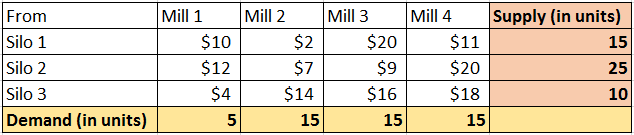Solution: Let’s understand what the above table explains.

The cost of transportation from Silo i to Mill j is given by the cost in each cell corresponding to the supply from each silo 1 and the demand at each Mill. For example, The cost of transporting from Silo 1 to Mill 1 is \$10, from Silo 3 to Mill 5 is \$18. It is also given the total demand & supply for mill and silos. The objective is to find the minimal transportation cost such that the demand for all the mills is satisfied.

As the name suggests Northwest corner method is a method of allocating the units starting from the top-left cell. The demand for Mill 1 is 5 and Silo 1 has a total supply of 15. So, 5 units can be allocated to Mill1 at a cost of \$10 per unit. The demand for Mill1 is met. then we move to the top-left cell of Mill 2. The demand for Mill 2 is 15 units, which it can get 10 units from Silo 1 at a cost of \$2 per unit and 5  units from Silo 2 at a cost of \$7 per unit. Then we move onto Mill 3, the northwest cell is S2M3. The demand for Mill 3 is 15 units, which it can get from Silo 2 at a cost of \$9 per unit. Moving on to the last Mill, Mill  4 has a demand of 15 units. It will get 5 units from a Silo 2 at a cost of \$20 per unit and 10 units from Silo 3 at a cost of \$18 per unit.

The total cost of transportation is = 5*10+(2*10+7*5)+9*15+(20*5+18*10) = \$520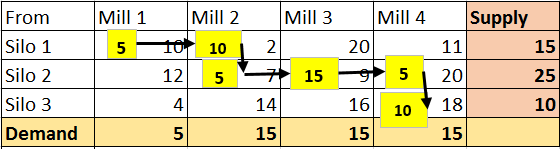### 6.2 Least Cost Method

Least Cost method is another method to calculate the most feasible solution for a linear programming problem. This method derives more accurate results than Northwest corner method. It is used for transportation and manufacturing problems. To keep it simple I am explaining the above transportation problem.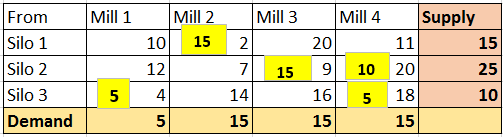According to the least cost method, you start from the cell containing the least unit cost for transportation. So, for the above problem, I supply 5 units from Silo 3 at a per-unit cost of \$4. The demand for Mill1 is met. For Mill 2, we supply 15 units from Silo 1 at a per unit cost of \$2. Then For Mill 3 we supply 15 units from Silo 2 at a per-unit cost of \$9. Then for Mill 4 we supply 10 units from Silo 2 at a per unit cost of \$20 and 5 units from Silo 3 an \$18 per unit. The total transportation costs are \$475.

Well, the above method explains we can optimize our costs further with the best method. Let’s check this using Excel Solver. Solver is an in-built add-on in Microsoft Excel. It’s an add-in plug available in Excel. Go to file->options->add-ins->select solver->click on manage->select solver->click Ok. Your solver is now added in excel. You can check it under the Data tab.

The first thing I am gonna do is enter my data in excel. After entering the data in excel, I have calculated the total of C3:F3. Similarly for others. This is done to take the total demand from Silo 1 and others.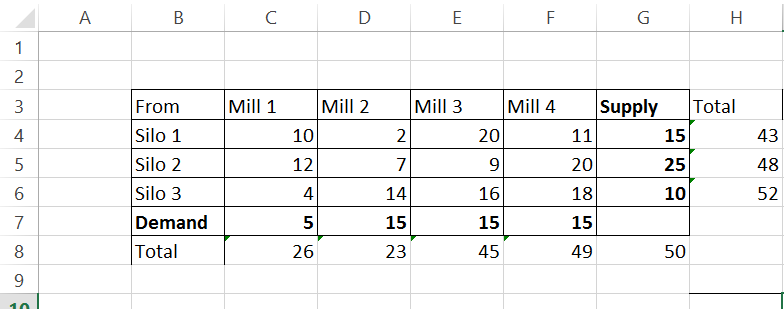After this, I am gonna break my model into two. The first table gives me the units supplied and the second table gives me the unit cost.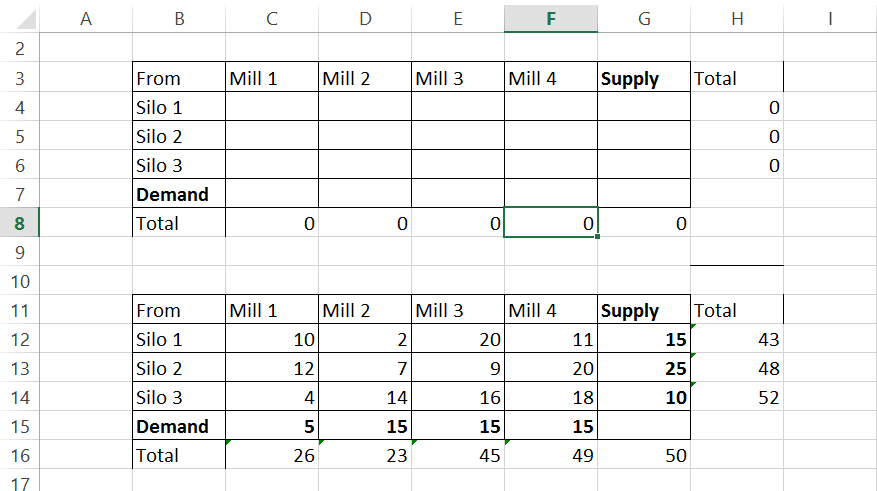Now, I am calculating my total cost which will be given by Sumproduct of unit cost and units supplied.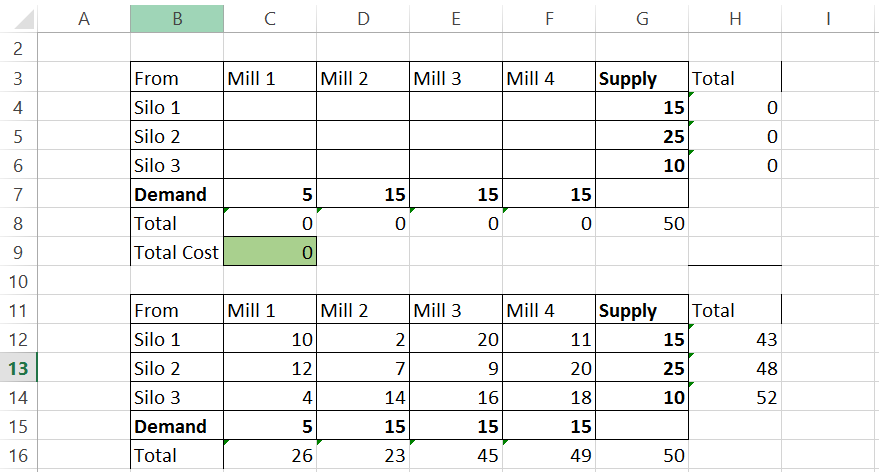Now I am gonna use Solver to compute my model. Similar to the above method. Add the objective function, variable cells, constraints.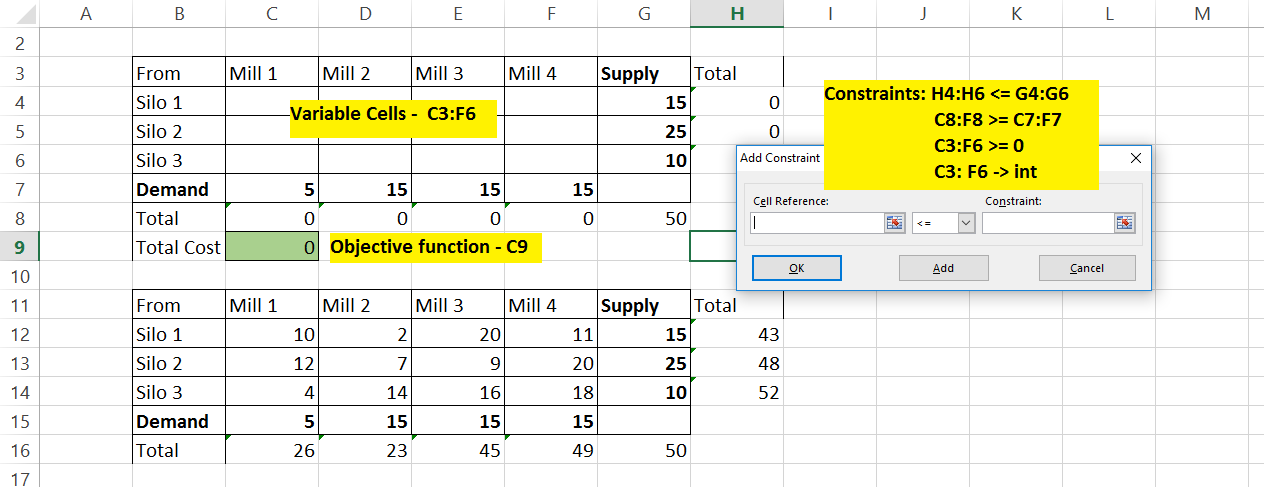Now your model is ready to be solved. Click on solve and you will get your optimal cost. The minimum transportation cost is \$435.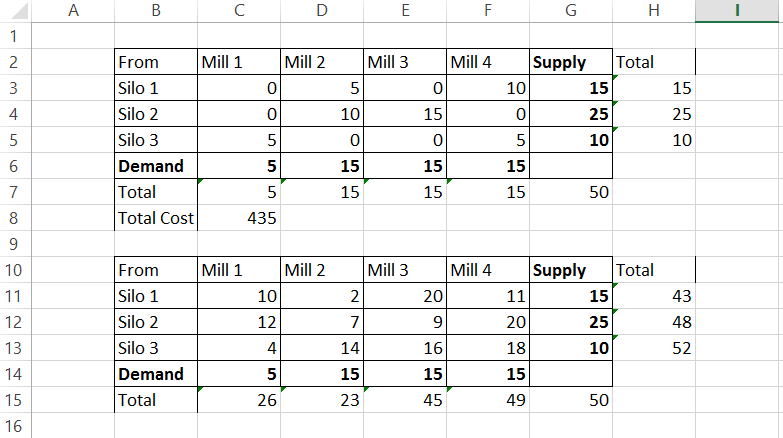## 7. Applications of Linear Programming

Linear programming and Optimization are used in various industries. The manufacturing and service industry uses linear programming on a regular basis. In this section, we are going to look at the various applications of Linear programming.

1. Manufacturing industries use linear programming for analyzing their supply chain operations. Their motive is to maximize efficiency with minimum operation cost. As per the recommendations from the linear programming model, the manufacturer can reconfigure their storage layout, adjust their workforce and reduce the bottlenecks. Here is a small Warehouse case study of Cequent a US-based company, watch this video for a more clear understanding.
2. Linear programming is also used in organized retail for shelf space optimization. Since the number of products in the market has increased in leaps and bounds, it is important to understand what does the customer want. Optimization is aggressively used in stores like Walmart, Hypercity, Reliance, Big Bazaar, etc. The products in the store are placed strategically keeping in mind the customer shopping pattern. The objective is to make it easy for a customer to locate & select the right products. This is subject to constraints like limited shelf space, a variety of products, etc.
3. Optimization is also used for optimizing Delivery Routes. This is an extension of the popular traveling salesman problem. The service industry uses optimization for finding the best route for multiple salesmen traveling to multiple cities. With the help of clustering and greedy algorithm, the delivery routes are decided by companies like FedEx, Amazon, etc. The objective is to minimize the operation cost and time.
4. Optimizations are also used in Machine Learning. Supervised Learning works on the fundamental of linear programming. A system is trained to fit on a mathematical model of a function from the labeled input data that can predict values from an unknown test data.

Well, the applications of Linear programming don’t end here. There are many more applications of linear programming in real-world like applied by Shareholders, Sports, Stock Markets, etc. Go on and explore further.

## End Notes

I hope you enjoyed reading this article. I have tried to explain all the basic concepts under linear programming. If you have any doubts or questions feel free to post them in the comments section. For easy understanding, we have broken this long article into a shorter course format – Linear Programming for Data Science Professionals

I have explained each concept with a real-life example. I want you to try them at your end and get hands-on experience. Let me know what you think!

## Learn, compete, hack and get hired!###### Analytics Vidhya

This is the official account of the Analytics Vidhya team.

## 25 thoughts on "Introductory guide on Linear Programming for (aspiring) data scientists"###### kumar says:February 28, 2017 at 5:43 am###### Swati Kashyap says:February 28, 2017 at 5:50 am###### Arun says:February 28, 2017 at 6:29 am
Nice Article. Well written !!! Reply###### Swati Kashyap says:February 28, 2017 at 6:33 am###### Dima says:February 28, 2017 at 7:40 am
Can you elaborate more on applications in Machine Learning? I was looking for uses of LP in ML, but all I found are rarely used applications - such as L1 norm-distance classifications and clustering. Reply###### Bipul says:February 28, 2017 at 8:21 am
Nice and simple explanation. And much needed for budding data scientist like me. Thanks! Reply###### JB says:February 28, 2017 at 11:21 am
Hi Swati, Thank you for your articles, they always teach useful stuff. Could you please provide printer versions of the article as I would like to collect them for later reference. Thank you Reply###### Swati Kashyap says:February 28, 2017 at 11:27 am
Hi JB, Thanks! And I'll look into it. Reply###### Swati Kashyap says:February 28, 2017 at 11:41 am
Hi Dima, Optimization is widely used in machine learning. For your better understanding, I'm sharing this link file:///C:/Users/lenovo/Documents/Optimization%20in%20Machine%20Learning.pdf Reply###### Geeta Shah says:February 28, 2017 at 1:32 pm###### Abhishek Kulkarni says:February 28, 2017 at 2:43 pm
Thank You for the insightful article. Always knew of the existence of optimization methods, but never of the applications. Reply###### Anil Muduli says:March 02, 2017 at 7:54 am
Very Good article with explanations. Appreciate it. Thanks. Reply###### Abhinandan Nuli says:March 02, 2017 at 2:35 pm
Amazing article!! Simple, yet effective one.. Thanks for sharing the info. Reply###### Anantha Sreenivas says:March 03, 2017 at 11:08 am
Hi Swati, Very informative and useful article, esp. with the real life examples. Thank you, Reply###### Rahul Ravipati says:March 08, 2017 at 4:34 am
Hi Swati, Great read. I studied Linear programming in my master's degree in industrial and systems engineering. I ahave know idea about data science.My doubt is how is linear programming used in data science? What is the relation between LP and data science? Reply###### Amit says:March 10, 2017 at 3:46 am
Nice article. Can you please provide the link to the document you are mentioning here. Would like to understand how optimization is used in machine learning environments. Reply###### Swati Kashyap says:March 10, 2017 at 7:47 am###### Amit says:March 10, 2017 at 7:52 am
Swati, this is the link to your local machine. I cant access it over the internet. Reply###### Swati Kashyap says:March 10, 2017 at 7:59 am###### Noor Mohamed M says:July 25, 2017 at 4:11 pm
it helped me to revise and quick wrap up the important concepts in operation research subject. Good work swati, Have you written anymore article. Reply###### Aviroop says:March 30, 2018 at 10:30 am
Great article Swati! AMPL and WinQSB are also great tools for solving LP problems. Reply###### Satnam says:April 01, 2018 at 9:19 pm
@Swati - Good effort. A suggestion - Use python-based libraries so that it can be integrated into the product as well. Also, venture into the BIP and MIP problems. Reply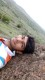###### SHUBHAM KSHIRSAGAR says:May 03, 2018 at 4:34 pm
BEST EXPLANATION!!! THANK YOU SO MUCH... Reply###### Thrishul says:May 17, 2018 at 7:53 pm